18529516814/0755-83206150/83258002#### What is Variable Frequency Driver(VFD)?

Rose   utmel.com   2021-10-21 17:37:00

 Topics covered in this article: Ⅰ. What is Variable Frequency Drive(VFD)? Ⅱ. The Classification of VFD Ⅲ. Control Mode for VFD Ⅳ. What are the Characteristics of a VFD?

## Ⅰ.What is Variable Frequency Drive(VFD)?

A variable frequency drive (VFD) is a power control device that uses power electrical technology to adjust the motor's operating power frequency and hence control the motor's speed. The main circuit's control is completed by the control circuit, the rectifier circuit transforms AC power to DC power, and the DC intermediate circuit influences the rectifier circuit. The inverter circuit converts direct current to alternating current, smoothing and filtering the output. A CPU for torque computation and some associated circuits are occasionally required for VFDs such as vector control frequency converters that require a significant number of calculations. Conversion of frequencies The goal of speed regulation is to do it by adjusting the frequency of the motor stator winding power supply. The rectifier circuit, buffer circuit, filter circuit, inverter circuit, and other circuits make up the VFD.

Rectifier circuit: It is primarily made up of a rectifier bridge, which converts alternating current (mains) to direct current via a complete bridge. After rectification, the theoretical DC voltage for three-phase 380V AC is 380X1.414537V; after rectification, the theoretical DC voltage for single-phase 220V AC is 220X1.414310V.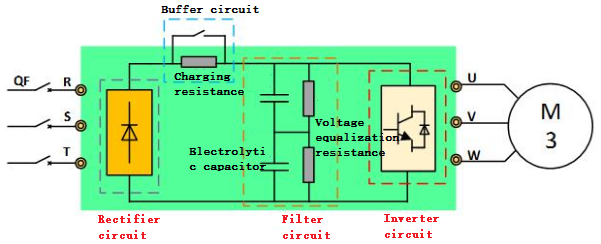Snubber circuit: reduces the inrush current when the power is turned on. When the VFD is turned on, the voltage across the capacitor does not change abruptly, but the current at both ends does. Both ends of the capacitor are comparable to a short circuit at this point. The rectifier bridge will be damaged by excessive current if there is no snubber circuit (charging resistor). The snubber circuit serves as a safeguard for the rectifier bridge.

Filter circuit: Electrolytic capacitors typically have a withstand voltage of 400V, while the three-phase 380V alternating current has a theoretical direct current voltage of roughly 537V after rectification. As a result, only two-stage electrolytic capacitors in series can be used to make the filter capacitor. Because the capacity of the electrolytic capacitors cannot be identical, the voltage distribution on the two-stage electrolytic capacitors after the series connection is imbalanced, causing the two electrolytic capacitors to have different service lives. To overcome the problem of unbalanced voltage, the two electrolytic capacitors must be connected in parallel with the same voltage-balancing resistors.

A power electronic circuit that converts direct current (DC bus) into the alternating current is known as an inverter circuit. The inverter bridge is made up of numerous IGBTs. Each IGBT contains a freewheeling diode, whose purpose is to provide a loop for the feedback energy of the motor's stator winding (motor power generation). When the motor generates electricity, the freewheeling diode allows the electric energy to flow into the DC circuit, charging the electrolytic capacitor.

## Ⅱ. The Classification of VFD

The frequency converter can be classified in a variety of ways. It can be divided into voltage type frequency converters and current type frequency converters based on the main circuit working mode; PAM control frequency converters, PWM control frequency converters, and high carrier frequency PWM control frequency converters based on the switch mode classification; and V/f control frequency converters based on the working principle. It can be classified as a general frequency converter, a high-performance special frequency converter, a high-frequency inverter, a single-phase inverter, or a three-phase inverter, depending on the application.

VVVF: Change the voltage and frequency. CVCF stands for constant voltage and frequency. The voltage and frequency of the AC power supply utilized in various nations, whether in homes or factories, are 400V/50Hz or 200V/60Hz (50Hz), respectively. A "frequency converter" is a device that converts an alternating current with a set voltage and frequency into alternating current with a variable voltage or frequency. The gadget converts the AC power from the power supply into direct current in order to generate variable voltage and frequency (DC).

Both voltage and frequency can be changed by the frequency converter utilized for motor control.

## Ⅲ. Control Mode for VFD

The output voltage of the low-voltage universal variable frequency output is 380650V, with an output power of 0.75400kW, a working frequency of 0400Hz, and an AC-DC-AC main circuit. Its control system has been passed down through four generations.

Control method using 1U/f=C sinusoidal pulse width modulation (SPWM).

It has a basic control circuit structure, is low cost, has good mechanical properties and hardness, and can meet the smooth speed regulation requirements of general transmission. It is widely utilized in many fields of industry. The torque is greatly affected by the stator resistance voltage drop when this control method is used at low frequency, due to the lower output voltage, which reduces the maximum output torque. Furthermore, its mechanical characteristics are not as difficult as those of a DC motor; its dynamic torque capacity and static speed regulation performance are not satisfactory, and the system performance is low; the control curve will change as the load changes; the torque response is slow, and the motor rotates. The torque utilization rate is low, and the performance suffers as a result of the stator resistance and the inverter's dead zone effect at low speeds, causing the stability to deteriorate. As a result, researchers have looked into vector control frequency conversion speed regulation.

Control method using voltage space vectors (SVPWM)

It is based on the premise of the three-phase waveform's overall generation effect, with the goal of approximating the ideal circular rotating magnetic field trajectory of the motor air gap, generating three-phase modulation waveforms all at once, and controlling in the manner of inscribed polygons approaching a circle. It has been improved after practical use, in that the addition of frequency compensation can eliminate speed control error; the amplitude of the flux linkage is estimated through feedback to eliminate the influence of stator resistance at low speeds, and the amplitude of the flux linkage is estimated through feedback to eliminate the influence of stator resistance at low speeds. To improve dynamic accuracy and stability, the output voltage and current are closed-loop. However, because the control circuit has many links and no torque adjustment is implemented, the system performance has not improved significantly.

Method of vector control (VC)

The three-phase to two-phase transformation is used to convert the asynchronous motor's stator currents Ia, Ib, Ic into the AC current Ia1Ib1 in the two-phase static coordinate system, which is then passed through the vector control variable frequency speed regulation method. It is equivalent to the DC current Im1 and It1 in the synchronous rotating coordinate system (Im1 is equivalent to the DC motor's excitation current; It1 is equivalent to the rotor magnetic field's directional rotation transformation). It1 imitates the DC motor's armature current proportional to torque), and then it1 is comparable to the armature current proportional to torque). The control approach is to acquire the DC motor's control quantity and use the related coordinate inverse transformation to control the asynchronous motor. The goal is to convert an AC motor to a DC motor and regulate the two components of speed and magnetic field separately. Orthogonal or decoupling control is achieved by managing the rotor flux and then decomposing the stator current to obtain the two components of torque and magnetic field via coordinate transformation. The introduction of the vector control approach is a game-changer. The introduction of the vector control approach is a game-changer. However, because the rotor flux linkage is difficult to accurately observe in practical applications, the motor parameters have a significant impact on the system characteristics, and the vector rotation transformation used in the equivalent DC motor control process is more complicated, making the actual control effect difficult to achieve the ideal analysis. result.

DTC (direct torque control) is a torque control method.

Professor DePenbrock of Ruhr University in Germany was the first to develop direct torque control frequency conversion technology in 1985. This technology has swiftly developed with unique control ideas, simple and transparent system structure, and great dynamic and static performance, and has largely solved the above-mentioned disadvantages of vector control. This technology is currently being used to power high-power AC drives for electric locomotive traction. Direct torque control examines the AC motor's mathematical model in the stator coordinate system and controls the motor's flux linkage and torque. It does not involve the conversion of an AC motor to a DC motor, which eliminates many difficult calculations in the vector rotation translation; it also does not require imitating the control of a DC motor or simplifying the AC motor's mathematical model for decoupling.

Control method using a matrix cross-cross matrix

AC-DC-AC frequency conversions include VVVF frequency conversion, vector control frequency conversion, and direct torque control frequency conversion. The input power factor is low, the harmonic current is high, the DC circuit necessitates a large energy storage capacitor, and the regenerative energy cannot be transmitted back to the grid, i.e., the four-quadrant operation is impossible. As a result, matrix AC-AC frequency conversion was invented. The huge and expensive electrolytic capacitor is eliminated because the matrix AC-AC frequency conversion eliminates the intermediary DC link.

——To achieve the speed sensorless mode, control the stator flux linkage and insert the stator flux linkage observer.

——In order to automatically identify motor parameters, automated identification (ID) relies on an accurate motor mathematical model.

——For real-time control, calculate the actual torque, stator flux linkage, and rotor speed corresponding to the stator impedance, mutual inductance, magnetic saturation factor, inertia, and so on.

——Make Band-Band control a reality. PWM signals are created to control the switching state of the inverter using Band-Band control of flux linkage and torque.

Matrix AC-AC frequency conversion features a quick torque response (two milliseconds), high-speed accuracy (2%, no PG feedback), and high torque accuracy (+3%); it also has a high beginning speed. Torque and high torque accuracy can generate 150 percent to 200 percent torque, especially at low speeds (even 0 speed).

## Ⅳ. What are the Characteristics of a VFD?

1. The soft-start feature

Start the motor with city power, with a starting current of 5-7 times the motor's rated current. The voltage of the power grid will drop when the motor is started immediately, affecting the regular operation of other electrical equipment.

The starting current of a frequency converter soft start is typically 1.2-1.5 times the rated current, thereby reducing the starting impulse current and the amount of transformer occupied.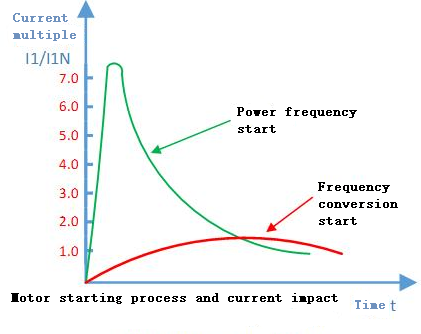2. Controlling the speed of frequency conversion

According to the same speed of the three-phase asynchronous motor, classical motor speed regulation is pole-changing speed regulation: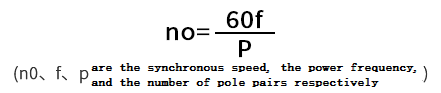The number of magnetic pole pairs in the stator winding of the motor can be altered to modify the synchronous speed of the motor, and hence the rotor speed. Two-pole, three-pole, and four-pole speeds can all be achieved using various combinations of winding connection methods. The speed range is limited, usually two speeds.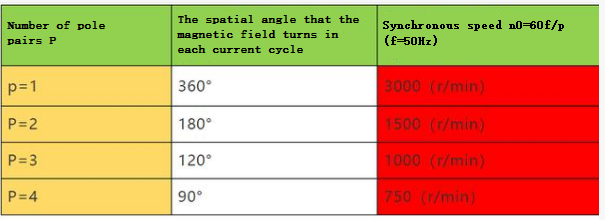Conversion of frequencies The relationship between the motor speed and the input frequency of the working power source is used to regulate speed: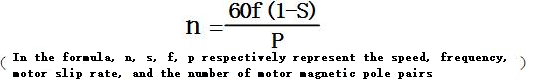Modifying the frequency f of the motor's operating power source achieves the goal of changing the motor's speed. The speed modification method is simple, and the motor may be set to various speeds depending on the process.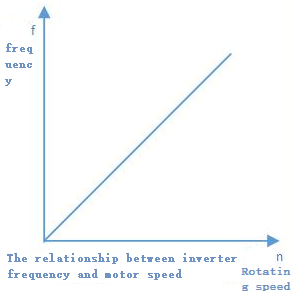3. The ability to save energy

The resistance generated by air or liquid is roughly proportional to the second power of the motor speed, and the necessary power is proportional to the third power of the motor speed in various fans, water pumps, and oil pumps.

Using the frequency converter to modify the air volume and flow when the needed air volume and flow are reduced can save a lot of electrical energy. The theoretical basis for power savings for pump loads is depicted in the diagram below.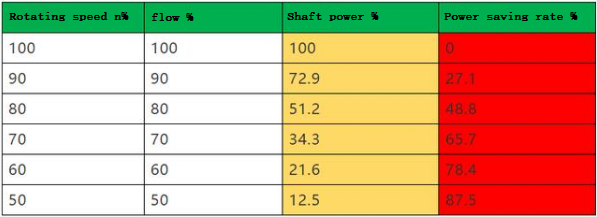18529516814/0755-83206150/83258002